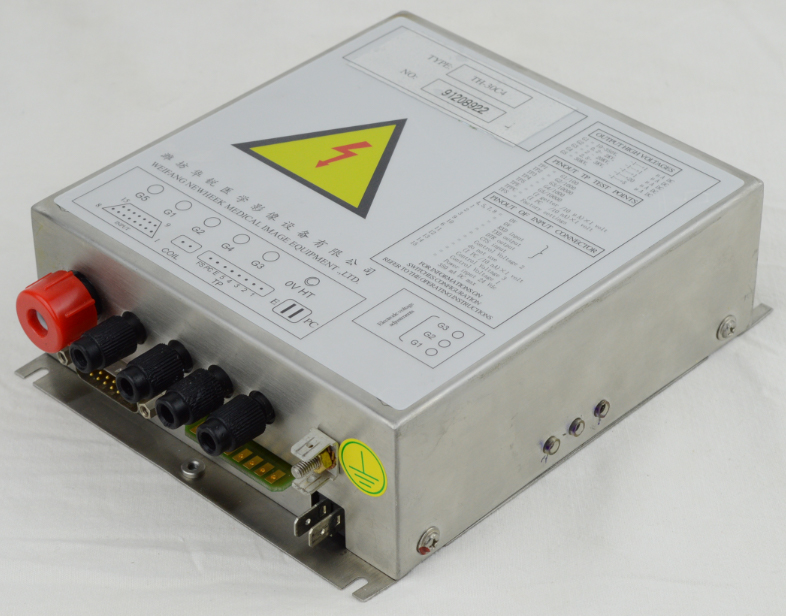﻿ Principle of high-voltage power supply - Professional Manufacturer Of X Ray Image Intensifier
Newheek specializes in producing X optical machine image intensifier, high voltage power supply,image intensifier TV system and so on.

# Principle of high-voltage power supply

When you work with high voltage power supply, it is beneficial to know the output drop and discharge time. Generally, we generate high voltage by controlling the inverter to feed the step-up transformer connected to the voltage multiplier circuit. The multiplier circuit (composed of capacitor and diode) uses this working principle: that is, the output of charging capacitor and discharging capacitor is the sum of series voltage of these capacitors every half cycle of AC voltage. The voltage multiplier circuit has a capacitive property and the ability to store and maintain charges.

In order to improve the efficiency, the resistance path connected to the internal output end of the power supply is a voltage feedback voltage divider resistance string with high impedance. The feedback voltage divider generates a voltage feedback signal of low voltage to ground reference to control and regulate the power supply.

Due to the direction of the diode in the multiplier device, the positive power supply can only provide current and cannot absorb current. Therefore, in the no-load state, the feedback voltage divider substring becomes the output discharge path. Let’s look at the multiplier capacitance value and feedback divider resistance value of a typical product to understand what kind of no-load discharge RC time constant we are talking about.

How long does the output discharge take under no-load condition. If the external load is connected to the output of the power supply, the discharge time constant can be greatly shortened. For this reason, the drop time of the high-voltage power supply is determined by the load. Remember this when you use high voltage power supply. If you have any needs, please call. Tel: 17616362242Author:Image Intensifier